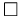# VASP relevant resources

VASP official forum

``` http://cms.mpi.univie.ac.at/vasp-forum/forum.php
```

VASP official wiki

``` http://cms.mpi.univie.ac.at/wiki/index.php/Main_page
```

Transition State Tools for VASP

```$\square$ Nudged Elastic Band: method for finding reaction pathways between two stable states.$\square$ Dimer: method for finding reaction pathways when only one state is known.$\square$ Lanczos: provides an alternative way to find the lowest mode and find saddle points.$\square$ Optimizers: provides an alternative way to find the lowest mode and find saddle points.$\square$ Dynamical Matrix: uses finite difference to find normal modes and reaction prefactors.
http://theory.cm.utexas.edu/vtsttools/$\square$ Useful scripts
http://theory.cm.utexas.edu/vtsttools/scripts.html
```

Dr. John Kitchin's DFT book (Modeling materials using density functional theory)

```$\square$ Abundant DFT and VASP experience sharing
http://kitchingroup.cheme.cmu.edu/dft-book/dft.html
```

WaveTrans: Real-space wavefunctions from VASP WAVECAR file (Dr. R. M. Feenstra and Dr. M. Widom)

```$\square$ WAVECAR visualization program by Fortran
http://www.andrew.cmu.edu/user/feenstra/wavetrans/
```

Dr. Sung Sakong's VASP plugin

```$\square$ For VMD
https://www.uni-due.de/~hp0058/?file=vmdplugins.html&dir=&lang=en$\square$ For Mathmatica
https://www.uni-due.de/~hp0058/?file=mathplugins.html
```

Dr. Byungki Ryu's Tips for VASP

``` https://sites.google.com/site/cta4rbk/home/tips4vasp
```

Dr. Ruifeng Zhang's learning

``` https://sites.google.com/site/zrftum/home/l
```

Dr. David Karhánek's Homepage

``` http://homepage.univie.ac.at/david.karhanek/downloads.html
```